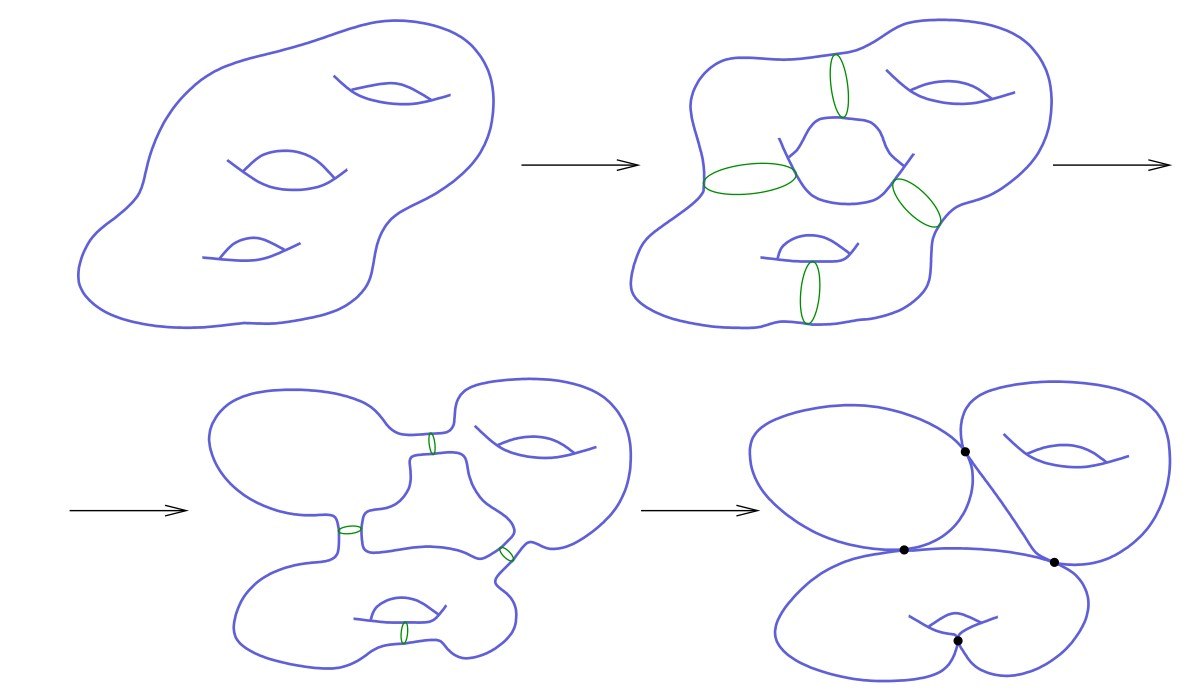## Vorlesung: Gromov-Witten Theory, Wintersemester 2022-23

### General information

Instructor: Prof. Chris Wendl (for contact information and office hours see my homepage)

Moodle:
https://moodle.hu-berlin.de/course/view.php?id=114268
The enrolment key is: enumerate
Important: You must join the moodle for the course in order to receive occasional time-sensitive announcements, e.g. if a lecture has been cancelled or rescheduled. HU students can access moodle using their HU username and password. Non-HU users can access it by following the above link and then setting up a HU Moodle Account with their external e-mail address as a username. You will need to enter the enrolment key printed above.

Time and place (newly revised as of 4.08.2022):
Lectures on Tuesdays 9:15-10:45 in room 1.012 and Wednesdays 15:15-16:45 in room 3.006 (RUD25)
Problem Class (Übung) Tuesdays 11:15-12:45 in room 1.012 (RUD25).

Language:
The course will be taught in English.Prerequisites:
I will assume that all students are comfortable with the essentials of differential geometry (smooth manifolds, vector fields and Lie bracket, differential forms and Stokes' theorem, de Rham cohomology, connections on vector bundles), as well as some algebraic topology (fundamental group, singular homology and cohomology) and functional analysis (continuous linear operators on Banach spaces, the standard Lp-spaces). Some previous knowledge of additional topics from topology (homological intersection theory, the first Chern class) and functional analysis or PDE theory (Fourier transforms, distributions, Sobolev spaces) will sometimes be helpful, though the relevant results can be taken as black boxes when necessary. For students who have not seen any symplectic geometry before, I will give a concise overview of the subject in the first one or two problem classes.

Contents:
Gromov-Witten theory lies in the intersection of three subbranches of mathematics: symplectic geometry, algebraic geometry, and mathematical physics. This course will focus mainly on the symplectic perspective, but it may also be of interest to students and researchers from the other two subjects.

Symplectic manifolds were invented around the turn of the 20th century as the natural geometric setting in which to study Hamilton's equations of motion from classical mechanics. The subject of symplectic geometry has developed considerably since then, and it retains a close connection with theoretical physics despite being technically a branch of “pure” mathematics. In particular, the subfield known as symplectic topology, which deals with “global” rather than “local” properties of symplectic manifolds, has witnessed an explosion of activity since the introduction of techniques from elliptic PDE theory in the 1980s. The most spectacular advances came from Gromov's theory of pseudoholomorphic curves, which has led to a wide assortment of algebraic invariants of symplectic manifolds, some of them related to structures that physicists study in quantum field theory or string theory. One example of this is the Gromov-Witten invariants, which are interpreted as counts of holomorphic curves satisfying specified constraints in a symplectic manifold. Since many interesting examples of symplectic manifolds are also algebraic varieties, the Gromov-Witten invariants are also heavily studied by algebraic geometers and can be viewed as a modern approach to enumerative problems (i.e. generalizations of the question “how many lines are there through two points?”) that have been studied in algebraic geometry since the 19th century.

The first goal of this course will be to establish the basic analytical underpinnings of the Gromov-Witten invariants: we will study the local and global structure of moduli spaces of Riemann surfaces and holomorphic curves, elliptic regularity theory for the nonlinear Cauchy-Riemann equation, Fredholm theory, the Riemann-Roch formula, transversality results via the Sard-Smale theorem, and Gromov's compactness theorem for pseudoholomorphic curves. These ingredients are sufficient to give a mathematically rigorous definition of the Gromov-Witten invariants for symplectic manifolds that satisfy a technical condition known as “semi-positivity”, which is always satisfied for manifolds of dimension at most six. Once this is established, there are various additional topics we might discuss, depending on the time available and interests of the class:

• The Kontsevich-Manin axioms for GW-invariants, and Kontsevich's recursion formula, which gives a beautiful answer to a fundamental question about the enumeration of rational curves in complex projective space.
• Quantum cohomology, a “deformation” of the usual cohomology ring of a manifold, in which the product depends on counts of holomorphic curves.
• Symplectic Calabi-Yau 6-manifolds, a setting in which each GW-invariant is determined by obstruction bundles over the moduli spaces of branched covers of finitely-many embedded curves.
• Advanced transversality methods: what can be done to define the GW-invariants for symplectic manifolds that are not semi-positive? (There are various approaches to this - I am likely to limit myself to the ones that I actually understand.)

Literature:
I will not be writing detailed lecture notes for this course, but will write up a brief summary of what was covered at the end of each week, including reading suggestions and exercises. A considerable amount of the material we'll cover is contained in notes that I have written for other courses in the past, notably:

The standard textbook for the construction of Gromov-Witten invariants on semi-positive symplectic manifolds is
It does not contain everything that we'll cover in the course, and does contain many things that we won't; in any case, anyone who gets serious about the subject will find it absolutely essential to acquire that book sooner or later. If you are new to symplectic geometry, you will find most of the basics explained nicely in
and you might also want to look at the introductory chapters of

Homework:
I will assign exercises sometimes. Sometimes I will discuss them in the problem class. They will not be graded.